• WORK
• TOOLS
• TRAINING
• VIEWS
• CONTACT

Scatterplot matrices are an excellent architecture for displaying large amounts of hypervariate data. Edward Tufte named this type of "multi-window plot" the most ingenious design. William Cleveland proposed giving an award to the (unknown) inventor of the scatterplot matrix and here is what he had to say about this graphical method:

"The inventor may not have fully appreciated the significance of the method or may have thought the idea too trivial to bring forward, but its simple, elegant solution to a difficult problem is one of the best graphical ideas around for displaying scattered measurements of three or more variables."

The basic concept of the scatterplot matrix is simple. Given a set of N variables, the scatterplot matrix is a N*N matrix containing all the pairwise scatterplots of the N variables in a single eye span—hence enabling comparisons.

Unfortunately, Excel charting library doesn't include native small multiples capabilities. So let’s build one in Excel. We're looking here at the famous Auto MPG data set. The data consist of three variables, that is, mpg, horsepower and acceleration measured for 398 cars. The matrix is made of 3*3 panels. Each panel of the matrix (except the diagonals) is a scatterplot of one variable against another.

The first step of the process is to create a scatterplot and add to it the vertical and horizontal grids that define the 9 panels. Since we have 3 panels in each direction we need to create four series—with 2 data points each—and crossing the axes at 1/3 (or 0.3333) and 2/3 (or 0.6666) as per the following setup:

It is important to constrain the minimum and maximum bounds for both the vertical and horizontal axis to 0 and 1 respectively. We also need to adjust the size of the scatterplot to have a square shape. Next, we need to set the lines of the grids to Solid Lines and their Marker options to None. The chart should look like this:

Next, we will add the pairwise data points to the matrix. But before doing that, we need to apply a transformation that changes the original data from its old scale to a new scale ranging between 0 and 1/3 (or 0.3333).

Take for instance the variable mpg. The values range between 9.0 and 46.6. If we consider a value X = 33.5 in this range and want to convert it to the new scale, then the transformation is as follows:

# HOW TO CREATE A SCATTERPLOT MATRIX IN EXCEL

HOW TO CREATE A SCATTERPLOT MATRIX IN EXCEL

Let's apply the above transformation to all the data points using the respective scaling factors for each variable as per the set up below.

You can see that the data points for mpg versus Horsepower has been transformed from the original scale to the new scale in order to fit within a square of size 1/3 = 0.33333.

The above chart looks great, doesn't it? But we still have a problem however. The scatterplot should be translated vertically by 1/3 to fit within the panel above. To do this we need to add 1/3 to every data point of the variable Horsepower. The chart should look like this after the translation:

Now lets add the scatterplot mpg versus Acceleration to the matrix. As expected the scatterplot is properly scaled but, again, is displayed in the wrong panel. The Acceleration data should be translated vertically by 2/3 to fit within the third panel above instead of being displayed on the first row.

To do this we need to add 2/3 = 0.6666 to every data point of the variable Acceleration. The chart should look like this after the translation:

Next, we add all the pairwise scatterplots—of the now scaled and translated data—to the matrix and, as you can see in the chart below, everything just fits in the right place.

There is one more problem however. The data is exactly fitting the 0.3333 square, and hence some data points just lay on the gridlines. To prevent that from happening we need to create a margin (say 10%) from the edges of the grids so that the data would fit into a smaller square than the 0.3333 one. Therefore, the transformation we've done earlier should be adjusted to take into consideration revised minimum and maximum values for the new scale as per the graph below:

The transformation above will shrink the footprint of the data from all sides of the grids by 10% and the chart will look like this:

And finally you can add the scales by following the same procedure used in this blog post while at the same time scaling and translating the data. With some formatting the chart could look like this :

Next, we need to add the labels of each variable to the diagonal panels of the matrix. To do that we need to create a new series with 3 data points. The data points are located at the center of each of the diagonal panels with x=y= 1/6 , 1/2 and 5/6 respectively as per the below graph. The trick is to set the Line and Marker options of the series to none and then add the data labels and set their Label Position option to Center.

Views > Excel charts > How to create a scatterplot matrix in Excel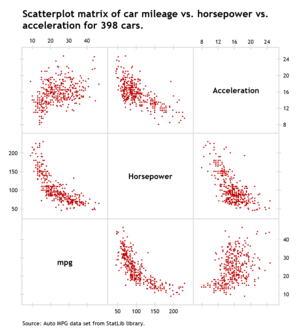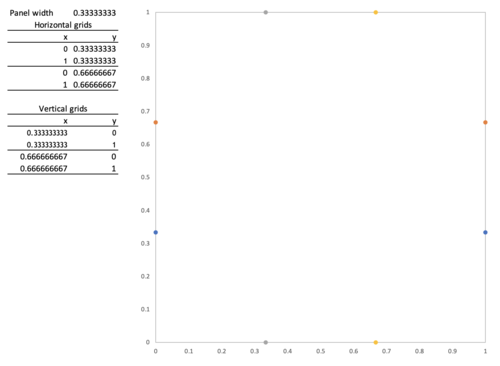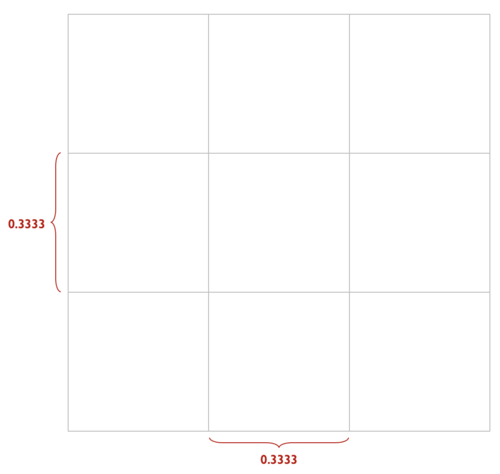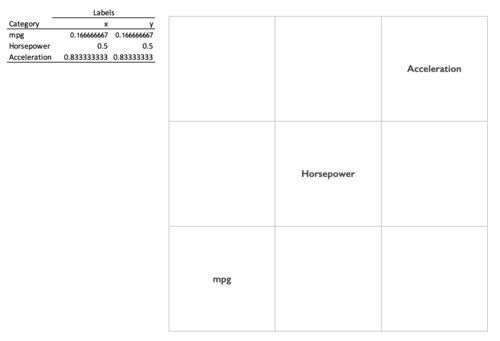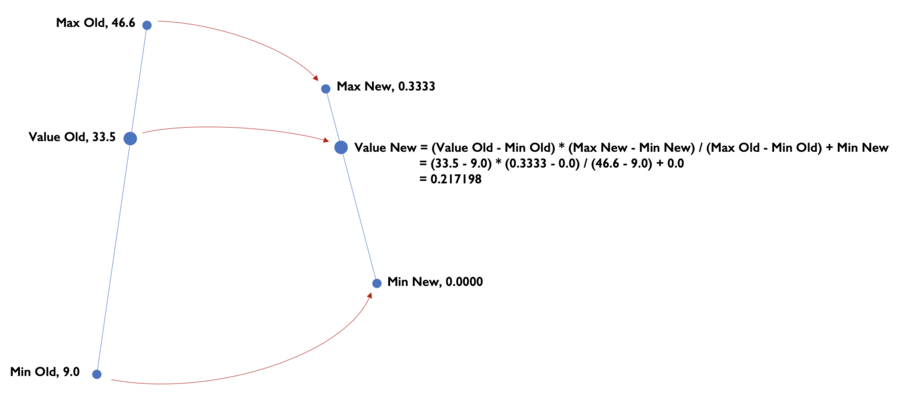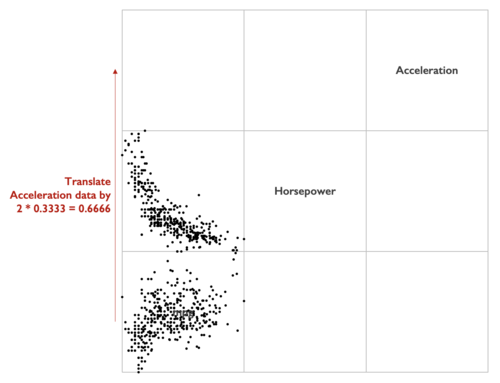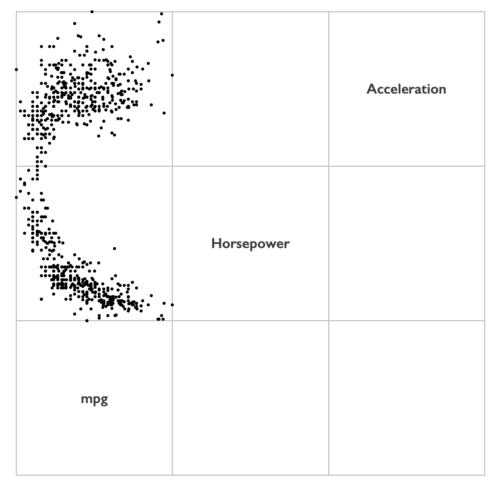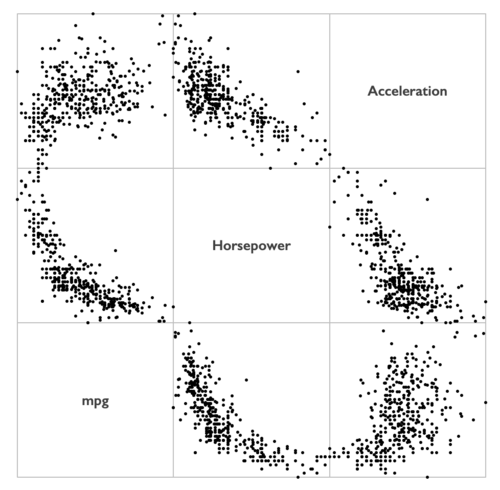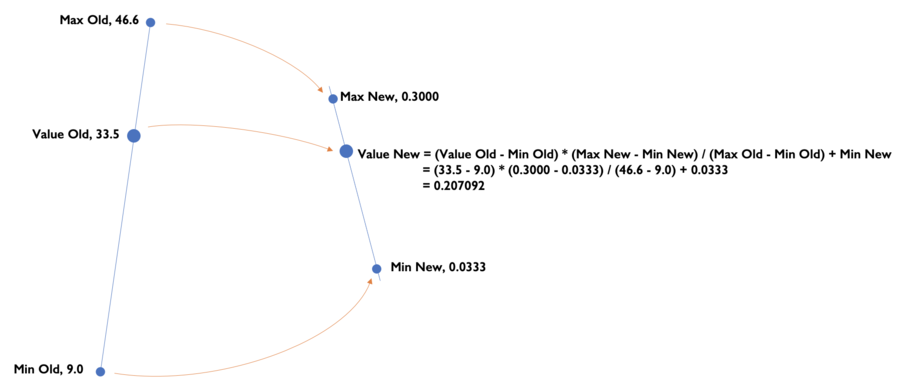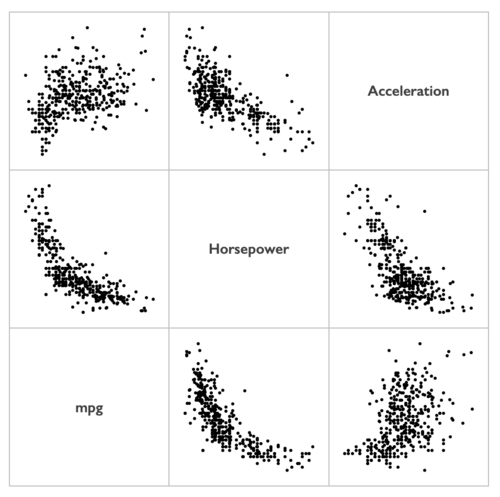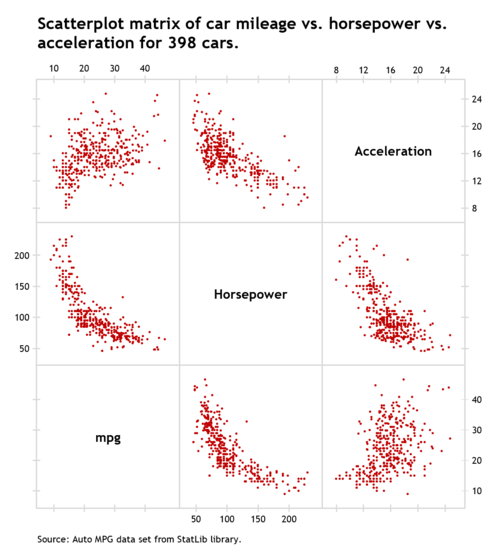Thank you for reading, and I hope you found this useful. If you have any questions, find me on Twitter and ask me anything.